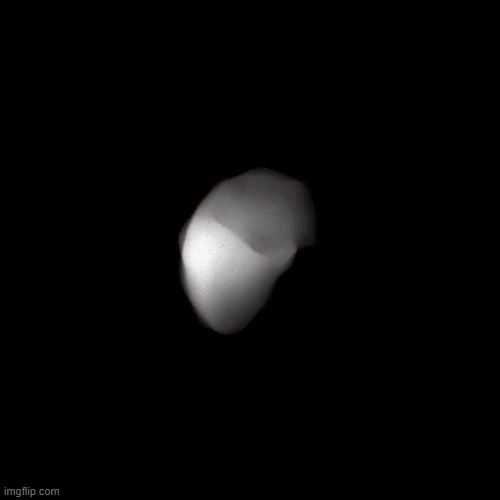# 3D point coordinates projection issue on the DRR image plane

I have implemented a code to get the corresponding 2D projection coordinate (i.e. x, y positions) of each vertex coordinate (i.e. 3D) on the DRR image projection plane.

But, I have a problem regarding this DRR projection code since it actually works for the projection angle zero, but if I project the same point cloud with a different angle say for example 90, then these projected points not aligned with the correct location in the corresponding DRR image.

In order to test this algorithm, I have first created a volumetric liver mesh and then voxelized it as a .mhd file to get the DRR images using SiddonJacobRay tracing algorithm  for different gantry rotations. Then I attempt to project the points of the liver mesh to each DRR images by providing the corresponding projection angle to the algorithm.

See the attached images for your further reference.

For this implementation I have referred links given below in addition to the link provided in .

I need your big help to resolve this issue.

For projection angle 0

For projection angle 36

For projection angle 72

Maybe take a look at this discussion about how to rotate an image?

I have applied changes according to the given link but still the error is there. Could you please let me know whether the above modifications are correct or not ? or do I need to apply this change to rotation parameters ?

The dummy CT scan which I have generated to obtain DRRs has a unit direction cosine matrix.
In my code, Euler 3D transform is used to rotate the volume to simulate the rotation of the linac gantry. The rotation is about z-axis.

According to the given link I have done below modifications to the code.

First I have updated m_Direction variable as below.

`m_Direction = itk.matrix_from_array(matrix_from_axis_angle(dtr * (-m_ProjectionAngle)))`

where matrix_from_axis_angle functionality is defiend as below. Here for teh ux, uy and uz I have used (0, 0, 1) respectively.

``````def matrix_from_axis_angle(angle):
""" Compute rotation matrix from axis-angle.
This is called exponential map or Rodrigues' formula.
Parameters
----------
R : array-like, shape (3, 3)
Rotation matrix
"""
ux = 0
uy = 0
uz = 1
theta = angle
#ux, uy, uz, theta = a
c = np.cos(theta)
s = np.sin(theta)
ci = 1.0 - c
R = np.array([[ci * ux * ux + c,
ci * ux * uy - uz * s,
ci * ux * uz + uy * s],
[ci * uy * ux + uz * s,
ci * uy * uy + c,
ci * uy * uz - ux * s],
[ci * uz * ux - uy * s,
ci * uz * uy + ux * s,
ci * uz * uz + c],
])

# This is equivalent to
# R = (np.eye(3) * np.cos(theta) +
#      (1.0 - np.cos(theta)) * a[:3, np.newaxis].dot(a[np.newaxis, :3]) +
#      cross_product_matrix(a[:3]) * np.sin(theta))

return R``````

In my code, Euler 3D transform is used to rotate the volume to simulate the rotation of the linac gantry. The rotation is about z-axis. I have implemented this code very similar to the syddonJacobRay tracing algorithm to project points on to the DRR image plane and set rotation parameters according to the this algorithm. I guess I miss something, but I’m not sure what it is.

This code actually works for only when the projection angle zero, otherwise not.

As you can see in the attached gif animation, the point cloud (see red dots) is rotate around wrong direction compared to the DRR images. How can I fix it ?Invert something? Rotate using the opposite angle? Or along axis (0,0,-1)?

I have no luck with the aforementioned changes.
I’m not able to see any difference between in my code compared to the SyddonJacob-ray-tracing algorithm, but there is an issue when changing the projection angle (angle > 0).

If possible can you please check my implementation ?

I highly appreciate your willingness to help me out regarding this matter. Thanks in advance for your time and consideration.